# To Do n In Lab tomorrow exams will

• Slides: 20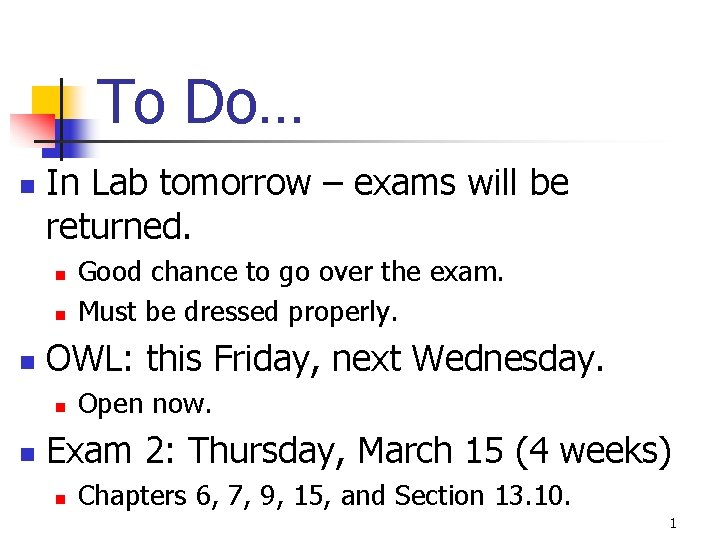To Do… n In Lab tomorrow – exams will be returned. n n n OWL: this Friday, next Wednesday. n n Good chance to go over the exam. Must be dressed properly. Open now. Exam 2: Thursday, March 15 (4 weeks) n Chapters 6, 7, 9, 15, and Section 13. 10. 1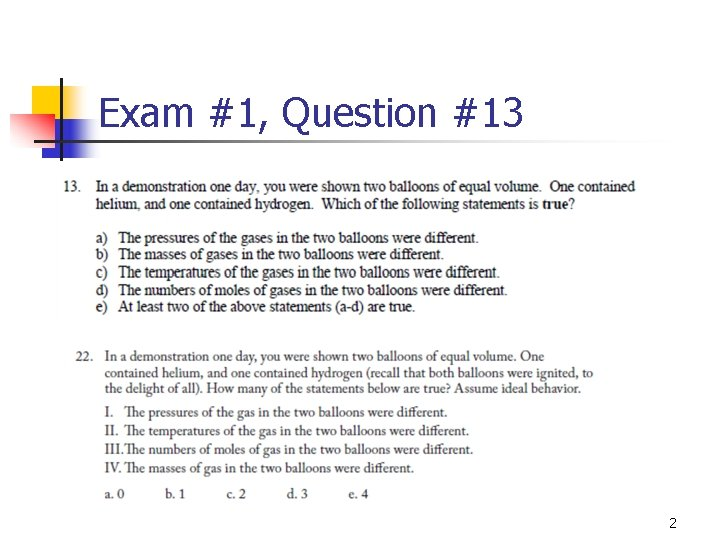Exam #1, Question #13 2Two Big Questions n n What information do we get from a balanced equation? What does it mean for an equation to be balanced? 3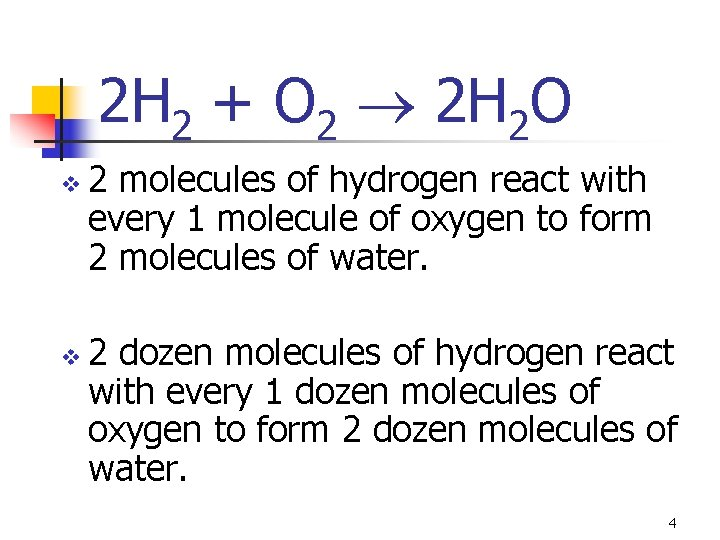2 H 2 + O 2 2 H 2 O v v 2 molecules of hydrogen react with every 1 molecule of oxygen to form 2 molecules of water. 2 dozen molecules of hydrogen react with every 1 dozen molecules of oxygen to form 2 dozen molecules of water. 4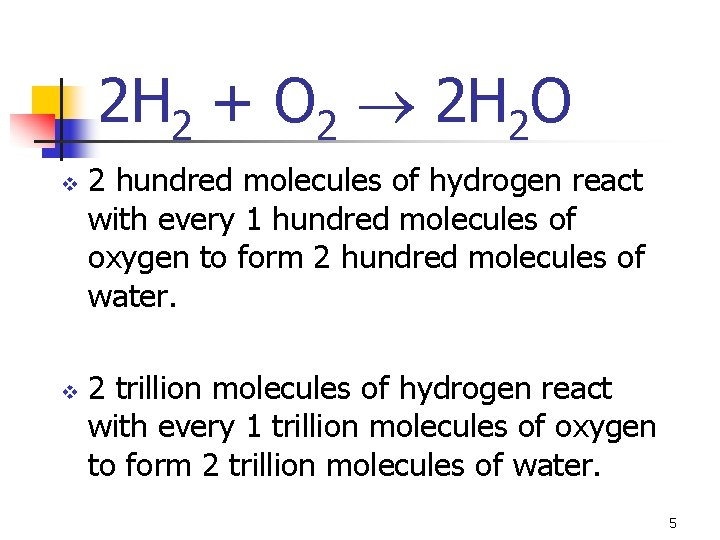2 H 2 + O 2 2 H 2 O v v 2 hundred molecules of hydrogen react with every 1 hundred molecules of oxygen to form 2 hundred molecules of water. 2 trillion molecules of hydrogen react with every 1 trillion molecules of oxygen to form 2 trillion molecules of water. 5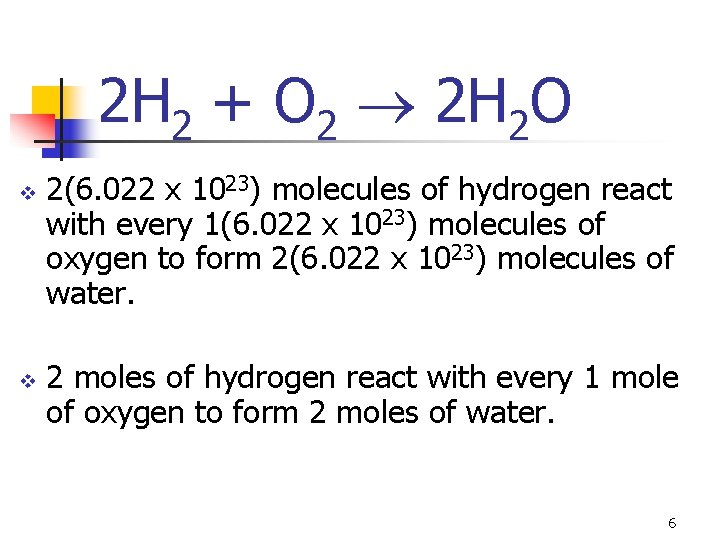2 H 2 + O 2 2 H 2 O v v 2(6. 022 x 1023) molecules of hydrogen react with every 1(6. 022 x 1023) molecules of oxygen to form 2(6. 022 x 1023) molecules of water. 2 moles of hydrogen react with every 1 mole of oxygen to form 2 moles of water. 62 H 2 + O 2 2 H 2 O v v 4 moles of hydrogen react with every 2 moles of oxygen to form 4 moles of water. 6 moles of hydrogen react with every 3 moles of oxygen to form 6 moles of water. 7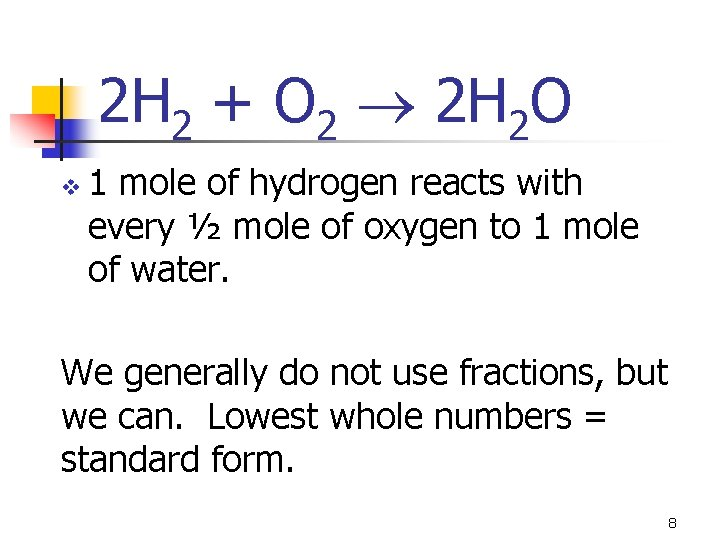2 H 2 + O 2 2 H 2 O v 1 mole of hydrogen reacts with every ½ mole of oxygen to 1 mole of water. We generally do not use fractions, but we can. Lowest whole numbers = standard form. 8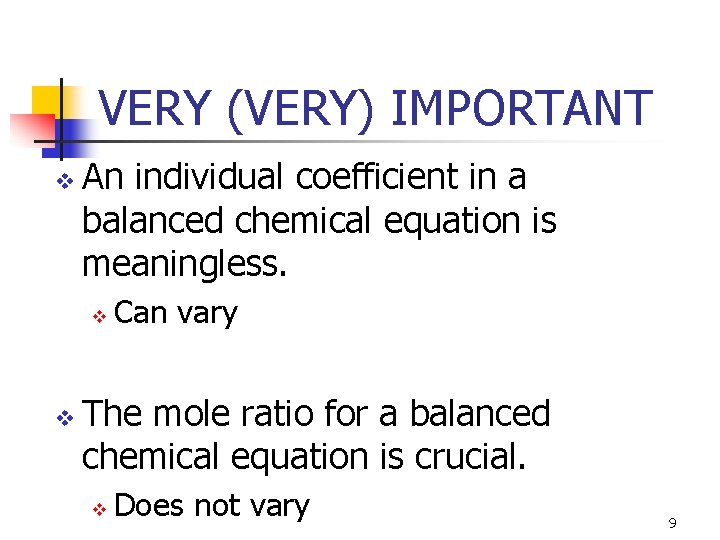VERY (VERY) IMPORTANT v An individual coefficient in a balanced chemical equation is meaningless. v v Can vary The mole ratio for a balanced chemical equation is crucial. v Does not vary 9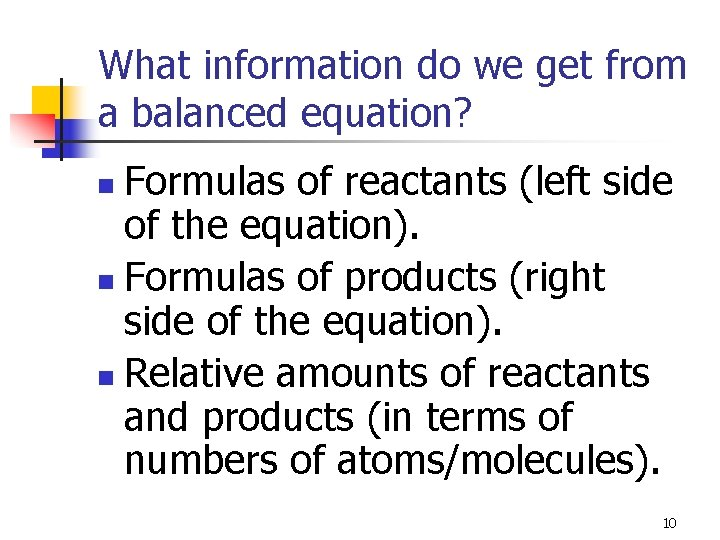What information do we get from a balanced equation? Formulas of reactants (left side of the equation). n Formulas of products (right side of the equation). n Relative amounts of reactants and products (in terms of numbers of atoms/molecules). n 10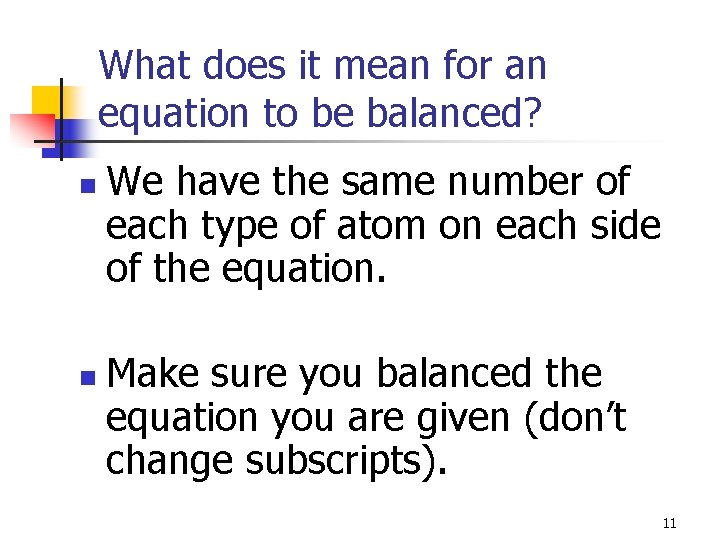What does it mean for an equation to be balanced? n n We have the same number of each type of atom on each side of the equation. Make sure you balanced the equation you are given (don’t change subscripts). 11Balancing Equations Educated trial and error is a good method. n Save the least complicated molecule for last. n 12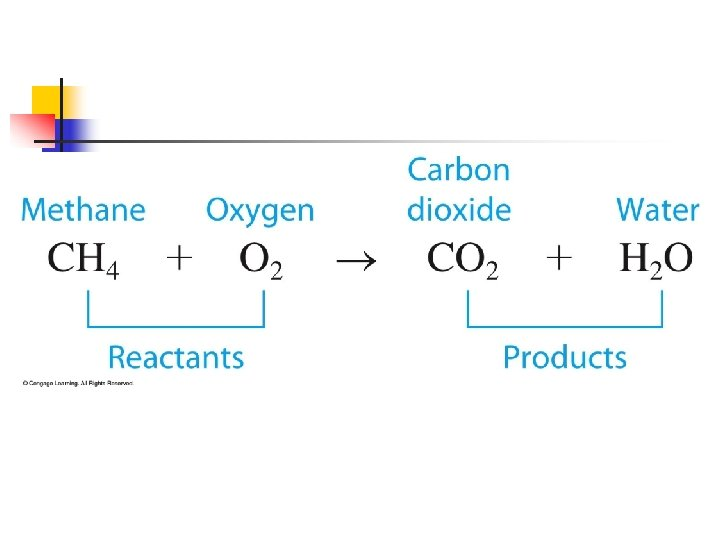Figure 6 -4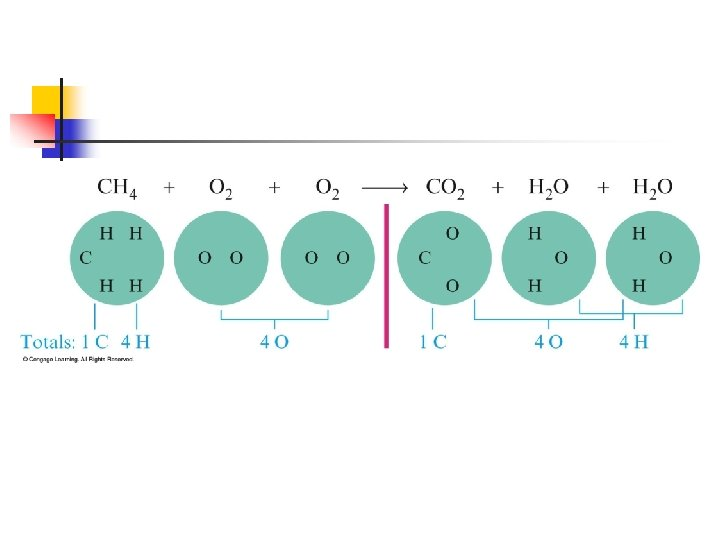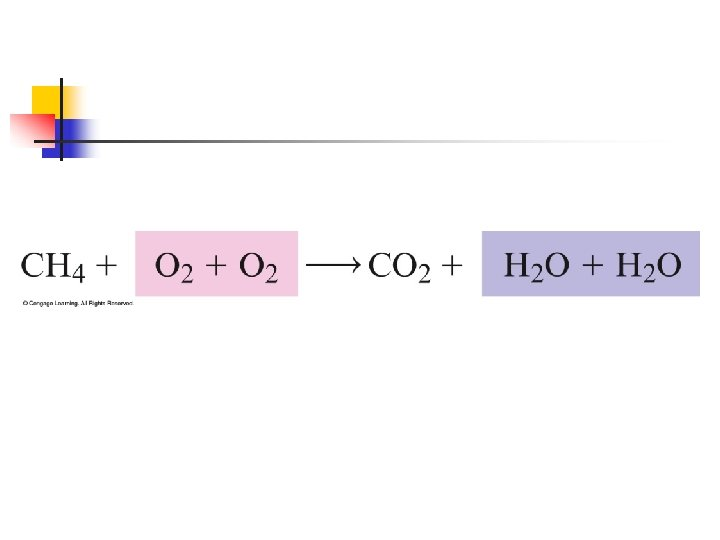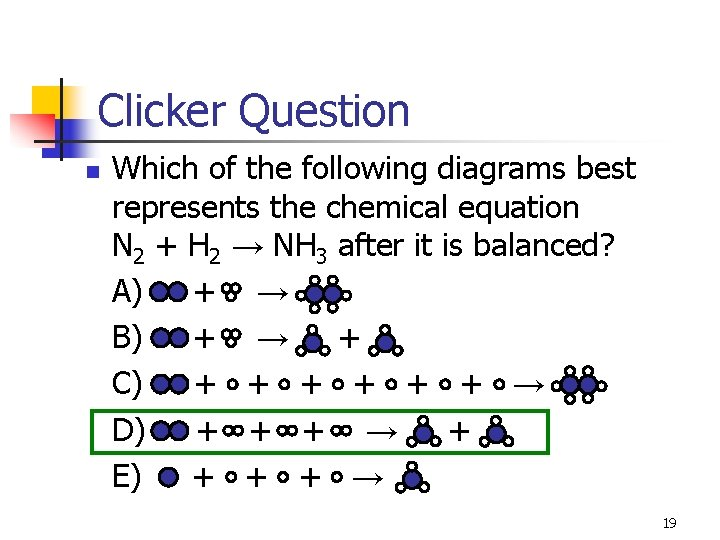Clicker Question n Which of the following diagrams best represents the chemical equation N 2 + H 2 → NH 3 after it is balanced? A) + → B) + → + C) + + + → D) + + + → + E) + + + → 19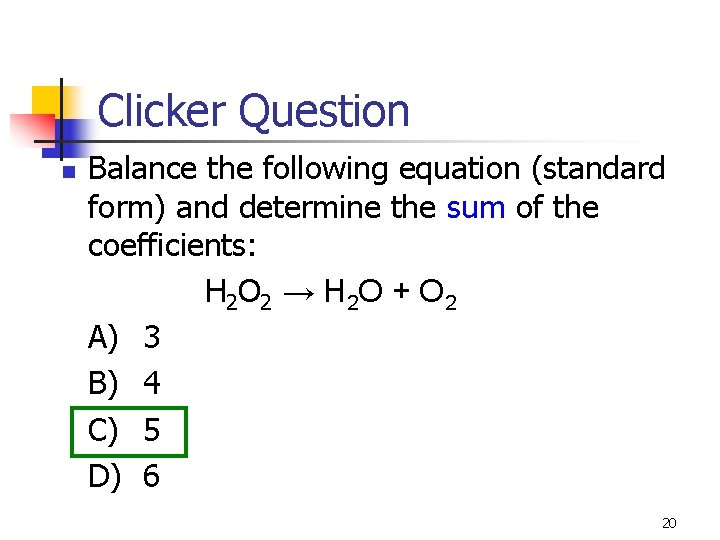Clicker Question n Balance the following equation (standard form) and determine the sum of the coefficients: H 2 O 2 → H 2 O + O 2 A) 3 B) 4 C) 5 D) 6 20